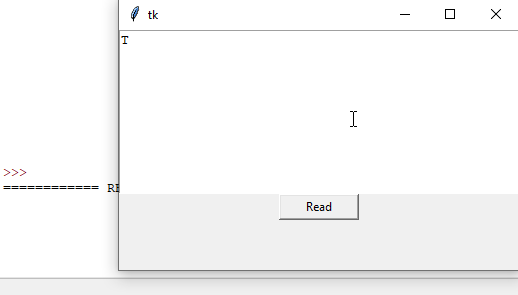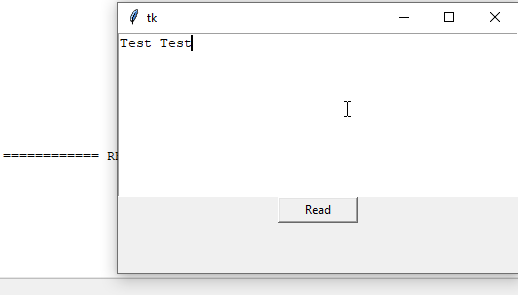# 如何获取 Tkinter 文本框中的输入

Tkinter `Text` 文本框控件具有 `get()` 方法以从文本框中返回输入，该文本框具有 `start` 位置参数和可选的 `end` 参数来指定要获取的文本的结束位置。

``````get(start, end=None)
``````

## 从 Tkinter 文本控件获取输入的示例代码

``````import tkinter as tk
root = tk.Tk()
root.geometry("400x240")

def getTextInput():
result=textExample.get("1.0","end")
print(result)

textExample=tk.Text(root, height=10)
textExample.pack()
btnRead=tk.Button(root, height=1, width=10, text="Read",
command=getTextInput)

btnRead.pack()

root.mainloop()
``````
``````result=textExample.get("1.0", "end")
``````

Tkinter 文本框控件中第一个字符的位置是 `1.0`，可以用数字 `1.0` 或字符串`"1.0"`来表示。

`"end"`表示它将读取直到文本框的结尾的输入。我们也可以在这里使用 `tk.END` 代替字符串`"end"``"end-1c"`表示位置是在`"end"`之前的一个字符。

## 从 Tkinter 文本控件最后获取不带`\n` 的输入的示例代码

``````import tkinter as tk
root = tk.Tk()
root.geometry("400x240")

def getTextInput():
result=textExample.get(1.0, tk.END+"-1c")
print(result)

textExample=tk.Text(root, height=10)
textExample.pack()
btnRead=tk.Button(root, height=1, width=10, text="Read",
command=getTextInput)

btnRead.pack()

root.mainloop()
``````## 相关文章 - Tkinter Text

• 如何使 Tkinter Text 控件变为只读